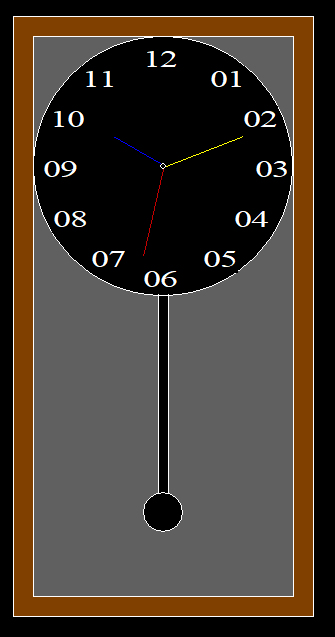Skip to content
Related Articles
C program to create a pendulum clock using graphics
• Last Updated : 23 Apr, 2021

In this article, it is discussed how to design a Pendulum Clock in the C programming language using Graphics.

Approach:

• Create two rectangles, one inside the other, using the rectangle() function to act as the outer outline of the clock & the other is the inner outline of the clock.
• Color the space between the two rectangles brown using setfillstyle() and floodfill() functions
• Implement a circle inside the inner rectangle using the circle() function
• Color all other parts leaving the circle with dark gray using setfillstyle() and floodfill() functions.
• In the circle, insert all the digits using settextstyle() and outtextxy() functions.
• Calculate the coordinates of the digits.
• Implement the pendulum with two lines by using the line() function & another circle by using the circle() function which will act as the bob.
• Color all of them black using the setfillstyle() and floodfill() functions again.
• Implement hour, minute & second hands by using the line() function.
• Color the objects individually by using the setcolor() function.

Below is the implementation of the above approach:

## C

 `// C program toc draw the pendulum clock ` `#include ` `#include ` `#include ` ` `  `// Driver Code ` `void` `main() ` `{ ` `    ``int` `gd = DETECT, gm; ` ` `  `    ``// Initialize of gdriver ` `    ``initgraph(&gd, &gm, ``"C:\\"` `                        ``"turboc3\\bgi"``); ` ` `  `    ``// Clock Outer Outline ` `    ``rectangle(500, 50, 800, 650); ` ` `  `    ``// Clock Inner Outline ` `    ``rectangle(520, 70, 780, 630); ` ` `  `    ``// Coloring Middle Part Of ` `    ``// Rectangle With Brown ` `    ``setfillstyle(SOLID_FILL, BROWN); ` `    ``floodfill(505, 55, 15); ` ` `  `    ``// Clock Outline ` `    ``circle(650, 200, 130); ` `    ``circle(650, 200, 3); ` ` `  `    ``// Coloring all the parts Of the ` `    ``// clock except the circle with ` `    ``// Darkgray ` `    ``setfillstyle(SOLID_FILL, DARKGRAY); ` `    ``floodfill(525, 355, 15); ` `    ``floodfill(522, 72, 15); ` `    ``floodfill(768, 72, 15); ` ` `  `    ``// Inserting Digits ` `    ``settextstyle(6, 0, 3); ` `    ``outtextxy(697, 100, ``"01"``); ` `    ``outtextxy(730, 140, ``"02"``); ` `    ``outtextxy(742, 190, ``"03"``); ` `    ``outtextxy(721, 240, ``"04"``); ` `    ``outtextxy(690, 280, ``"05"``); ` `    ``outtextxy(630, 300, ``"06"``); ` `    ``outtextxy(578, 280, ``"07"``); ` `    ``outtextxy(540, 240, ``"08"``); ` `    ``outtextxy(530, 190, ``"09"``); ` `    ``outtextxy(537, 140, ``"10"``); ` `    ``outtextxy(569, 100, ``"11"``); ` `    ``outtextxy(630, 80, ``"12"``); ` ` `  `    ``// Left Line Of Pendulum ` `    ``line(645, 328, 645, 528); ` ` `  `    ``// Right Line Of Pendulum ` `    ``line(655, 328, 655, 528); ` ` `  `    ``// Pendulum Bob ` `    ``circle(650, 546, 20); ` ` `  `    ``// Coloring Line & Bob With Black ` `    ``setfillstyle(SOLID_FILL, BLACK); ` `    ``floodfill(652, 544, 15); ` `    ``floodfill(647, 330, 15); ` ` `  `    ``// Creating the Hour Hand ` `    ``// & Color Blue ` `    ``setcolor(BLUE); ` `    ``line(647, 197, 600, 170); ` ` `  `    ``// Creating Minute Hand ` `    ``// & Color Yellow ` `    ``setcolor(YELLOW); ` `    ``line(653, 200, 730, 170); ` ` `  `    ``// Creating Second Hand and the ` `    ``// Color Red ` `    ``setcolor(RED); ` `    ``line(650, 203, 630, 290); ` ` `  `    ``// Hold the screen for a while ` `    ``getch(); ` ` `  `    ``// Close the initialized gdriver ` `    ``closegraph(); ` `} `

Output:Want to learn from the best curated videos and practice problems, check out the C Foundation Course for Basic to Advanced C.

My Personal Notes arrow_drop_up
Recommended Articles
Page :